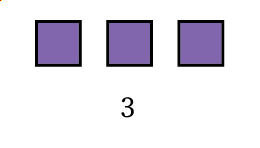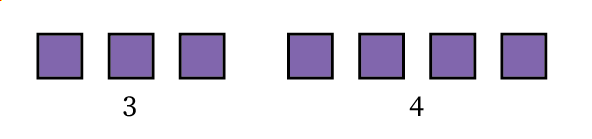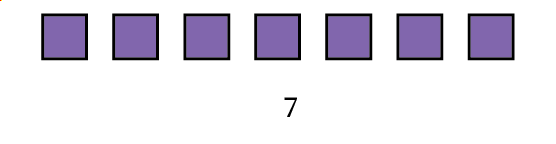## Notation and Modeling Addition of Whole Numbers

### Learning Outcomes

• Use symbols and words to describe addition of single and double digit numbers
• Model addition of single and double digit numbers using base-10 blocks, or shapes

A college student has a part-time job. Last week he worked $3$ hours on Monday and $4$ hours on Friday. To find the total number of hours he worked last week, he added $3$ and $4$.

The operation of addition combines numbers to get a sum. The notation we use to find the sum of $3$ and $4$ is:

$3+4$

We read this as three plus four and the result is the sum of three and four. The numbers $3$ and $4$ are called the addends. A math statement that includes numbers and operations is called an expression.

To describe addition, we can use symbols and words.

Operation Notation Expression Read as Result
Addition $+$ $3+4$ three plus four the sum of $3$ and $4$

### example

After talking to her counselor, Esmeralda needs to determine how many classes she has taken so far at Blue Sky College. Last year she took $6$ classes, and this year will complete $8$ classes. She needs to determine the sum of $6$ and $8$. First translate the sentence into math notation and then calculate the results.

Translate from words to math: $6+8$

The operation of addition combines numbers to get a sum

We read this as six plus eight and the result is the sum of six and eight.

$6+8=14$

## Model Addition of Whole Numbers

Addition is really just counting. We will model addition with $\text{base - 10}$ blocks. Remember, a block represents $1$ and a rod represents $10$. Let’s start by modeling the addition expression we just considered, $3+4$.
Each addend is less than $10$, so we can use ones blocks.

 We start by modeling the first number with 3 blocks.Then we model the second number with 4 blocks.Count the total number of blocks.There are $7$ blocks in all. We use an equal sign $\text{(=)}$ to show the sum. A math sentence that shows that two expressions are equal is called an equation. We have shown that. $3+4=7$.

### example

Model the addition $2+6$.

### try it

Model: $3+6$.

Model: $5+1$.

When the result is $10$ or more ones blocks, we will exchange the $10$ blocks for one rod.

### example

Model the addition $5+8$.

Notice that we can describe the models as ones blocks and tens rods, or we can simply say ones and tens. From now on, we will use the shorter version but keep in mind that they mean the same thing.

### try it

Model the addition: $5+7$.

Model the addition: $6+8$.

Next we will model adding two digit numbers.

### example

Model the addition: $17+26$.

### try it

Model each addition: $15+27$.

Model each addition: $16+29$.

Watch the video below for another example of how to use base $10$ blocks to model the sum of two whole numbers.